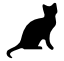## Grade 5 - Percentages

### Choose correct answer(s) from given choice

1)Sarah has $3401 with and spends 22 % of it on groceries. How much money is left with her?  a.$ 2752.78 b. $2652.78 c.$ 748.22 d. $2952.78 2)A man donated 8% of his salary to charity and spent 12% of the remaining. He still has$ 13398.88 left. What was his total salary?
 a. $16550 b.$ 17520 c. \$ 16400 d. none of these
3)A cat is walking on periphery of a regular polygon as shown below.If it starts from point s, cat will be on which side after walking 94% distance of the periphery in clock-wise direction?
 a. B b. A c. F d. G

### Fill in the blanks

 4) 45% of 20% of 800 =
 5) The chart shows production of different apparel in a garment factory. If production of suits is 10500 pieces, the total production will be pieces6) squares need to be un-shaded to cover 75% of the total area.7) Jason got 60 % in his Computer test. If the test was of a total of 50 marks, then his score was
8)Convert the fraction representation of the percentages given below into the decimal form:

 13 3 20
% =

 75 1 4
% =

 25 3 20
% =

 61 1 20
% =
 9) Betty stays in the school hostel and gets Rs. 5432 from home every month. She spends 22 percent of this amount on hostel fees. The monthly hostel fees is Rs.

### Check True/False

 10) 49% of 55% of 1500 = 55% of 49% of 1500 True    False